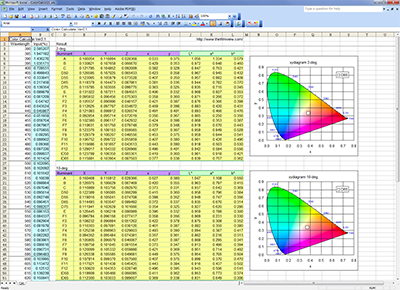# Simple calculation tools

## Convert relative reflection to absolute reflection

Convert relative reflectance to absolute reflectance for spectrophotometer measured value.

1. Measurement method:
Normal (Reference=100)
Reverse (Sample=100) When set the reference and the sample vice versa.

2. Reference glass:

3. Wavelength(nm):

4. Measurement value (Relative reflectance):

Result (Absolute reflectance):

Excel version is here: reltoabs.xls

## Substrate Index

### Calculate the refractive index of a substrate without absorption

Calculate refractive index of substrate from reflectance or transmittance of non-coated substrate.

1. Measurement method:
One side reflectance
Both side reflectance (Both side polish surface substrate)
Both side transmittance (Both side polish surface substrate)

2. Measurement value(%):

Result (Refractive index of substrate):

Excel version is here: substindexcalc.xls

### Calculates the refractive index, absorption coefficient, and internal transmittance of a substrate with absorption

Excel version is here: Subst_nkTi_Calc.xlsx

## Mean Free Path

Calculate Mean Free Path.

1. Kind of gas:

2. Temperature(Celsius, ℃):

3. Pressure(Pa):

Input format: 0.001 or 1e-3

Result:
Mean Free Path (m)
Mean Velocity (m/s)
Impingement rate (pcs/s*m2)

References: 真空技術実務読本 オーム社, Japan

## Color Calculation

Calculate the color system values from XYZ tristimulus values.

1. Observer:

2. Illuminant:

3. XYZ:
X:
Y:
Z:

Result:
x , y

L* , a* , b*
, C* , h

Hunter L , a , b

L* , u* , v*
, u' , v'

sRGB R , G , B

### Calculate XYZxy L*a*b* from spectrum values. (Excel sheet)

colorcalc-v30.xlsxJuly 13, 2020 Fixed an error in the calculation of L*a*b* when the value of X/Xn, Y/Yn, Z/Zn was 0.008856 or less. (Excel sheet only).